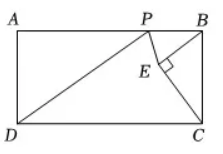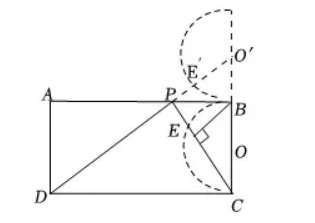$\text{A.}$ 8 $\text{B.}$ $4 \sqrt{5}$ $\text{C.}$ 10 $\text{D.}$ $4 \sqrt{5}-2$

A

#### 解析：

【解析】解: 设点 $O$ 为 $B C$ 的中点, 由题意可知, 点 $E$ 在以 $B C$ 为直径的半圆 $O$ 上运动, 作半圆 $O$ 及线段 $B C$ 关于 $A B$ 的对称图形 (半圆 $\left.O^{\prime}\right)$, 点 $O$ 的对称点为 $O^{\prime}$, 点 $E$ 的对称点为 $E^{\prime}$, 连接 $O^{\prime} E^{\prime}, P E^{\prime}$, 则 $P E=P E^{\prime}$,\begin{aligned} & \therefore D O^{\prime}=10, \\ & \text { 又 } \because O^{\prime} E^{\prime}=2, \\ & \therefore D E^{\prime}=D O^{\prime}-O^{\prime} E^{\prime}=8 \text {, 即 } P D+P E \text { 的最小值为 } 8 . \end{aligned}